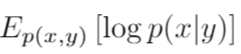# Get KL-divergence for CSIS

Hi,

I am new to Pyro and wondering how we can get the exact value from the objective function (expected forward KL divergence) when using Compiled Sequential Importance Sampling (CSIS). If I am understanding correctly, the objective function of CSIS is

(from the paper Inference Compilation and Universal Probabilistic Programming) and the `loss` term from Pyro CSIS implementation is evaluating the first term, which excludes the constant term. I am wondering how can we evaluate the constant term and get the exact value of the objective function, which is the expected forward KL-divergence?

I think the constant is the value ofHow can we evaluate it?